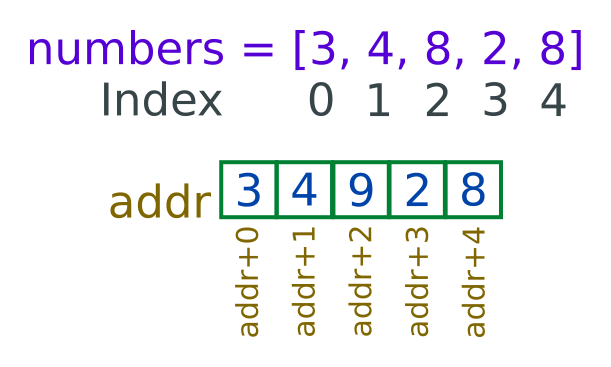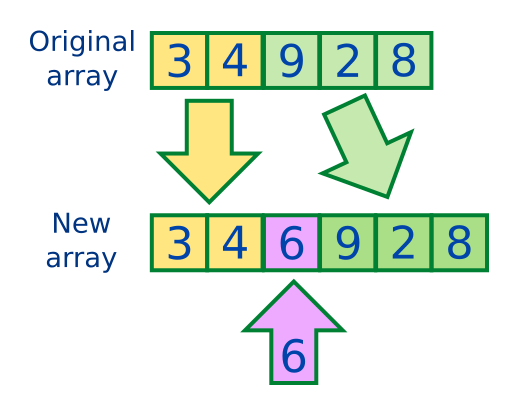# Arrays

Martin McBride, 2018-05-04
Tags array abstract data type
Categories data structures

An array is a type of data structure that holds an ordered list of data values. It is very useful if you need to keep a set of related values together.

Most programming languages feature arrays, and they usually work in a similar way. We will use pseudocode to illustrate arrays typically work, using the ARRAY keyword. The details may vary slightly between different real languages.

## Declaring an array

You can declare an array like this:

```ARRAY days = ["Sun", "Mon", "Tue", "Wed", "Thu", "Fri", "Sat"]
```

This creates an array called days which contains 7 strings, representing the short names of the days of the week.

## Accessing individual elements

The values in the array are called ''elements''. You can access an individual element using square brackets. Elements are numbered starting from 0, so:

• days is the first element, "Sun".
• days is the second element, "Mon".
• days is the third element, "Tue".

The following code would print Sun, Tue, Fri:

```PRINT(days)
PRINT(days)
PRINT(days)
```

## Modifying elements

You can set the elements in an array using assignment, like this:

```days = "First"
days = "Last"
```

The array would now contain:

```["First", "Mon", "Tue", "Wed", "Thu", "Fri", "Last"]
```

## Looping through the elements

You can use a for loop to loop through all the elements in an array, like this:

```FOR i = 0 TO 6
PRINT(days[i])
ENDFOR
```

Notice that the loop counter i starts at 0 and counts up to one less than the length of array (ie 6).

## Why does the index start at 0?

In most languages, the array index start at 0. This seems a bit odd at first, but you get used to it and it often makes things easier. Consider this array, numbers:

```numbers = [3, 4, 9, 2, 8]
```

The basic reason the index starts at 0 is that the elements of an array are stored contiguously (next to each other) in memory. In the array numbers, if addr is the memory address of the start of the array, then:

• The first element is at addr + 0.
• The second element is at addr + 1.
• The third element is at addr + 2.So the index in the array is also the offset from the start of the array to the required element.

## Arrays size is static

The arrays we are discussing in this topic are static arrays. This simply means that you have to decide how long the array is when you create it. Once you have created an array of a particular length, you cannot change its length. Many languages provide static arrays.

The alternative to a static array is a dynamic data structure usually called a list. Lists are more flexible than arrays, for example you can increase their size after they have been create. The downside is that they can be slightly slower, and use a bit more memory.

## Inserting a value into an array

What if we wanted to insert a number 6 at position 2 in our numbers arrays. So instead of:

```[3, 4, 9, 2, 8]
```

we would have:

```[3, 4, 6, 9, 2, 8]
```

The main problem is that our existing array only has 5 elements, and we are trying to create an array with 6 elements. What is the solution?

Well we must create a brand new array with 6 elements. Then we need to copy the 5 elements from the old array into the new array - but leaving a gap at the second element. Finally we copy the new value, 6, into the second element.We have a similar problem if we want to delete an element from an array, or join two arrays together. If you find that you need to do this type of thing in your program, you should probably be using lists instead of arrays. They have this functionality already built in, using optimised code.